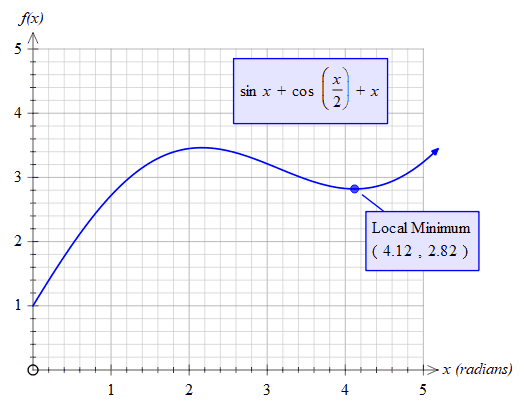﻿ fnMinVal

# fnMinVal

Top  Previous  Next
 fnMinVal(function, low, high)   Finds the minimum y value  between low and high. For example:fnminval(sinx+cos(x/2)+x,2,5) = 2.82 fnminval(sinx+cos(x/2)+x,0,1) = 0   To find the x value of the local minimum, use the fnmin function